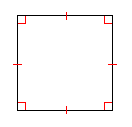# Squares: Area and Perimeter

A square is a rectangle with four congruent sides. A square can also be defined as a rhombus with four right angles.The perimeter $P$ of a square is given by the formula

$P=4s$ ,

where $s$ is the length of a side.

The area $A$ of a square is given by the formula

$A={s}^{2}$ .